﻿

### Isomorphisms between lattices of nearly normal subgroups

#### Abstract

A subgroup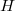$H$ of a group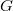$G$ is said to be nearly normal in$G$ if it has a finite index in its normal closure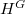$H^G$. The set nn(G) of nearly normal subgroups of$G$ is a sublattice of the lattice of all subgroups of$G$. Isomorphisms between lattices of nearly normal subgroups of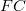$FC$-soluble groups are considered in this paper. In particular, properties of images of normal subgroups under such an isomorphism are investigated. Moreover, it is proved that if$G$ is a supersoluble group and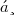$Ḡ$ is an$FC$-soluble group such that the lattices nn(G)and nn(Ḡ) are isomorphic, then also Ḡ is supersoluble.

DOI Code: 10.1285/i15900932v20n1p43

Keywords: Nearly normal subgroup; Lattice isomorphism

Classification: 20F24

Full Text: PDF